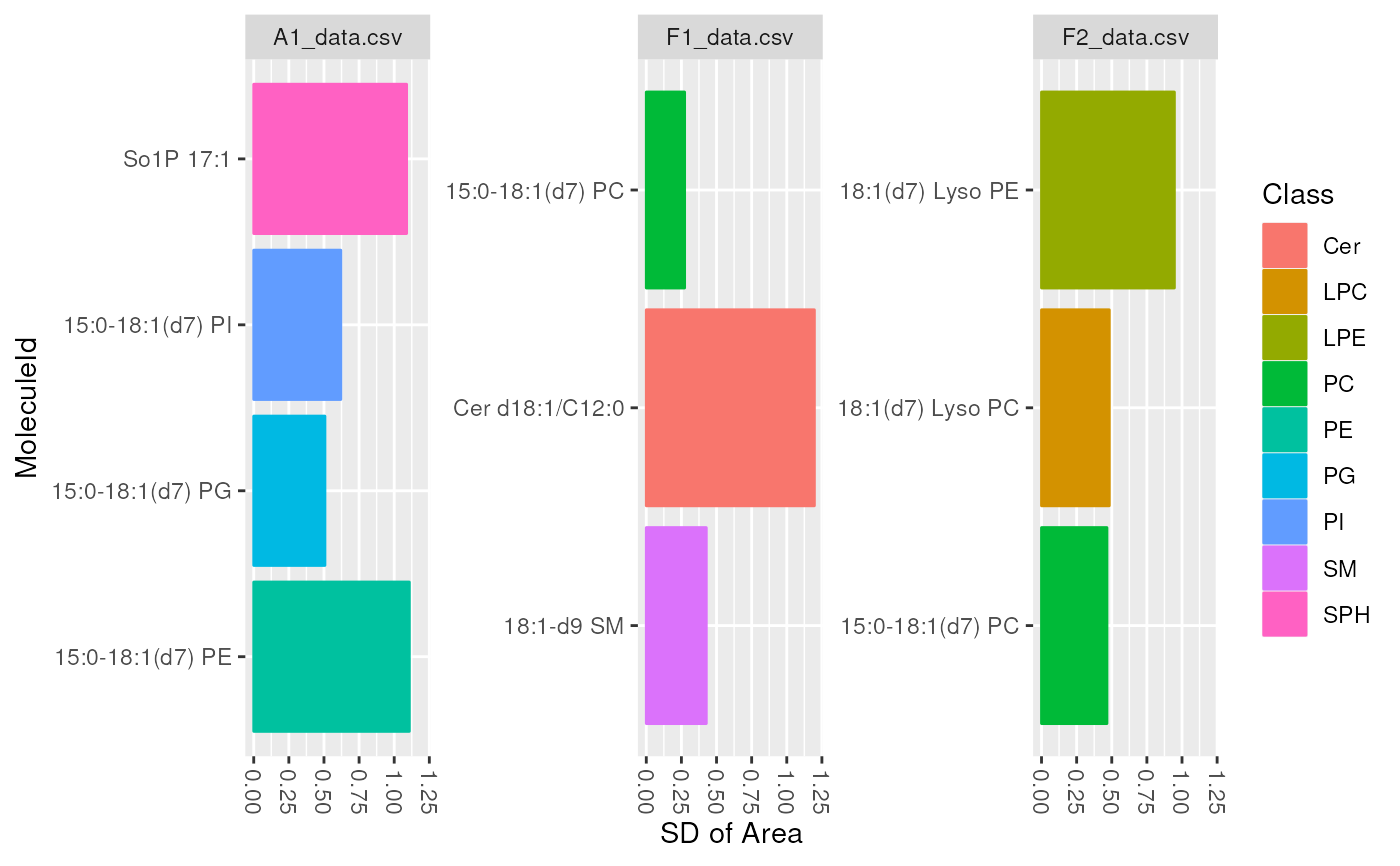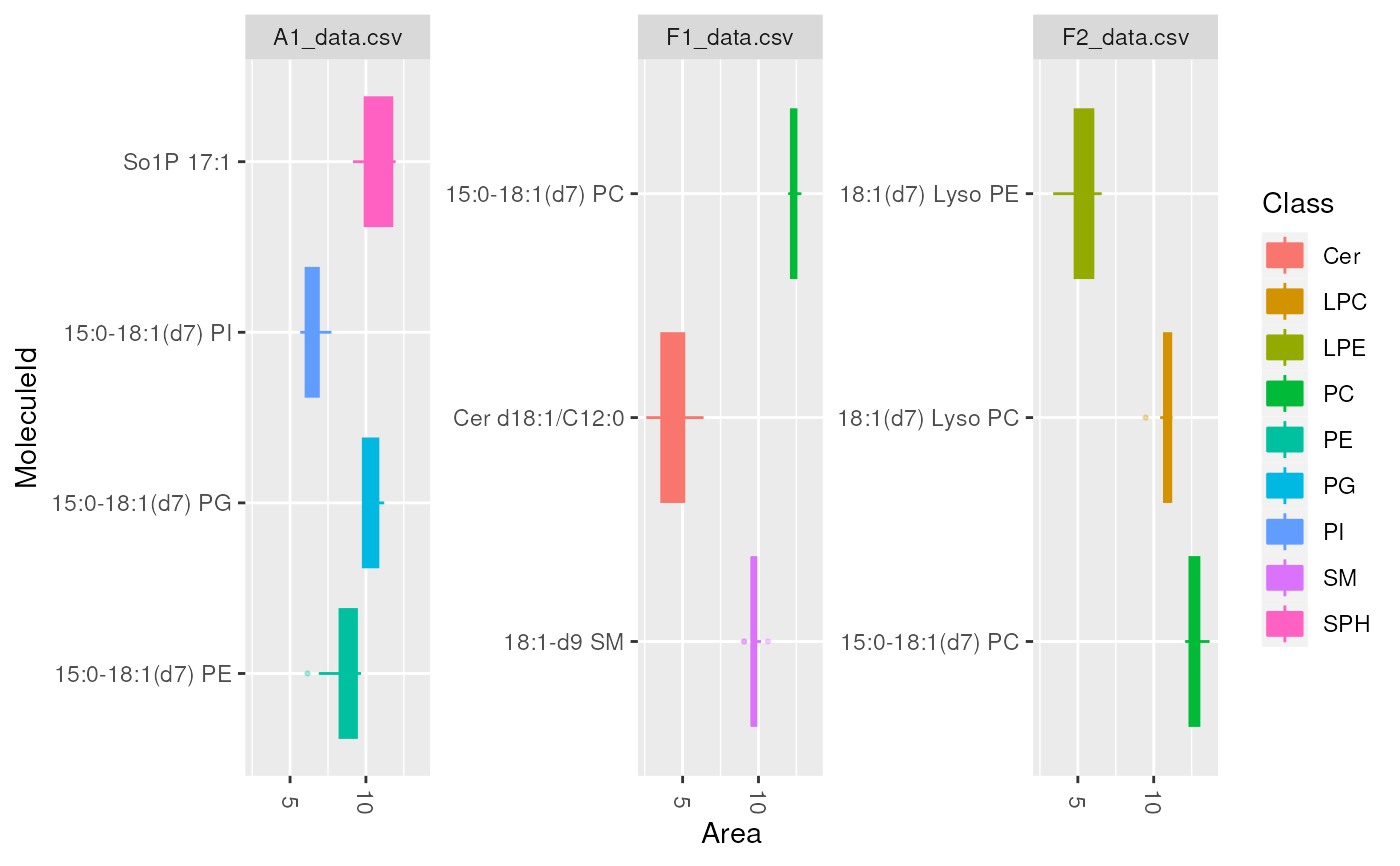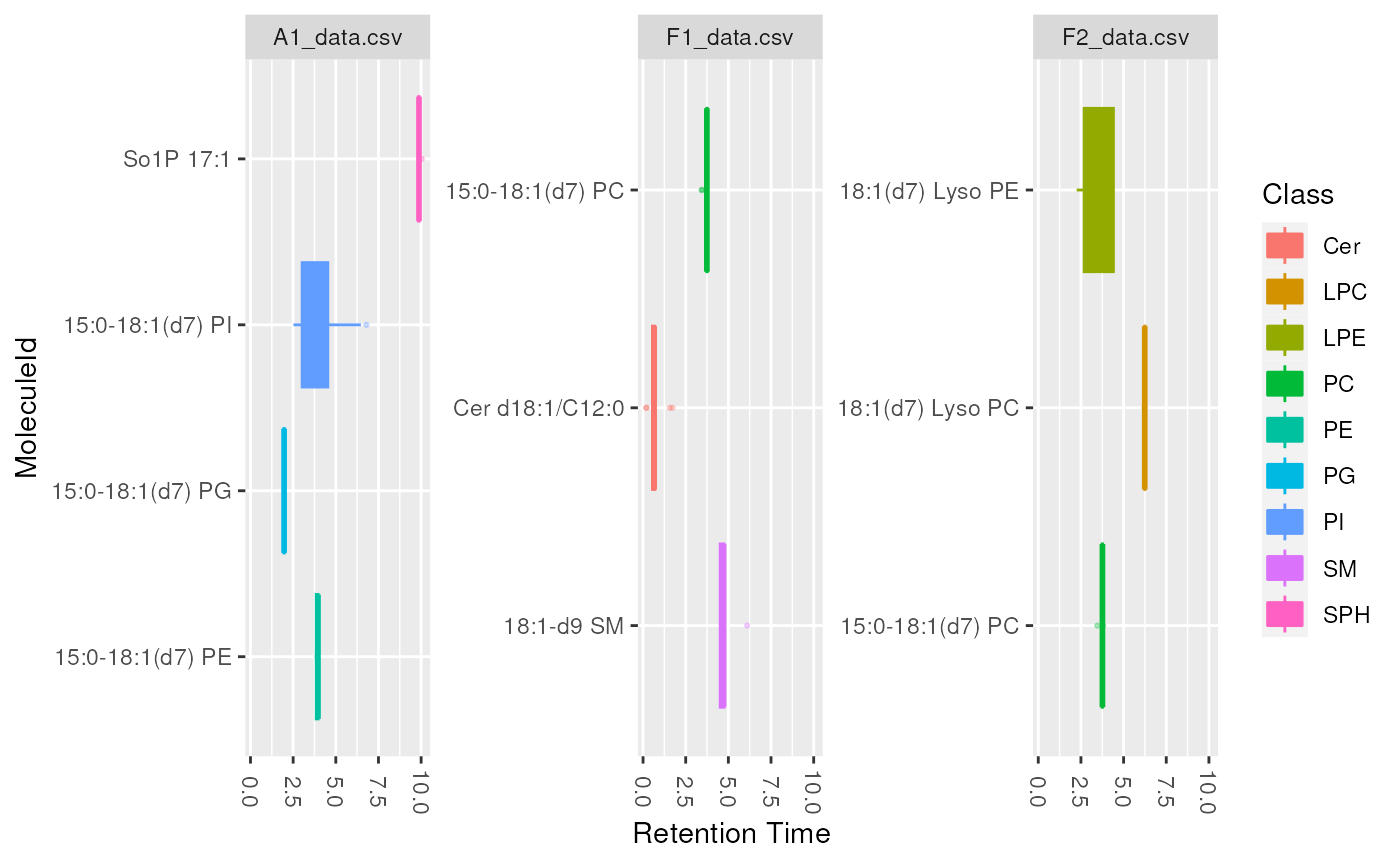lipidr supports three types of plots for to visualize at lipid molecules.

cv plots a bar chart for coefficient of variation of lipid molecules. This plot type is usually used to investigate the CV in lipid intensity or retention time, in QC samples.

sd plots a bar chart for standard deviations of a certain measure in each lipid. This plot type is usually used to look at standard deviation of intensity for each lipid, but can also be used to look at different measures such as Retention Time, to ensure all lipids elute within expected range.

boxplot plots a boxplot chart to examine the distribution of values per lipid. This plot type is usually used to look at intensity distribution for each lipid, but can also be used to look at different measures, such as Retention Time or Background.

plot_molecules(
data,
type = c("cv", "sd", "boxplot"),
measure = "Area",
log = TRUE,
color = "Class"
)

## Arguments

data

LipidomicsExperiment object.

type

plot type, either cv, sd or boxplot. Default is cv.

measure

Which measure to plot the distribution of: usually Area, Area Normalized or Height. Default is Area

log

Whether values should be log2 transformed (Set FALSE for retention time). Default is TRUE

color

The column name of a row annotation to be used as color

A ggplot object.

## Examples

data(data_normalized)
d_qc <- data_normalized[, data_normalized$group == "QC"] # plot the variation in intensity and retention time of all measured # lipids in QC samples plot_molecules(d_qc, "cv", "Area")plot_molecules(d_qc, "cv", "Retention Time", log = FALSE) #> Warning: Removed 1 rows containing non-finite values (stat_summary).# plot the variation in intensity, RT of ISTD (internal standards) # in QC samples d_istd_qc <- data_normalized[ rowData(data_normalized)$istd,
data_normalized\$group == "QC"
]
plot_molecules(d_istd_qc, "sd", "Area")plot_molecules(d_istd_qc, "sd", "Retention Time", log = FALSE)
#> Warning: Removed 1 rows containing non-finite values (stat_summary).plot_molecules(d_istd_qc, "boxplot")plot_molecules(d_istd_qc, "boxplot", "Retention Time", log = FALSE)
#> Warning: Removed 1 rows containing non-finite values (stat_boxplot).